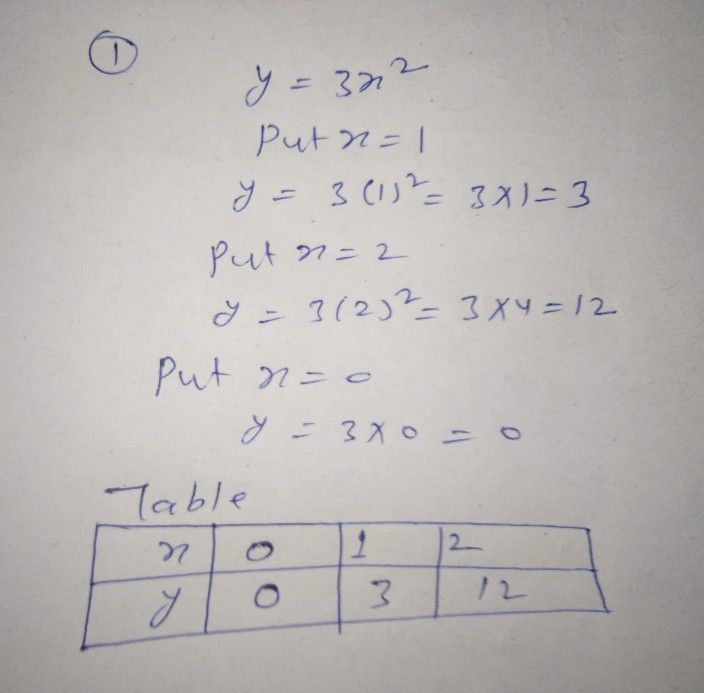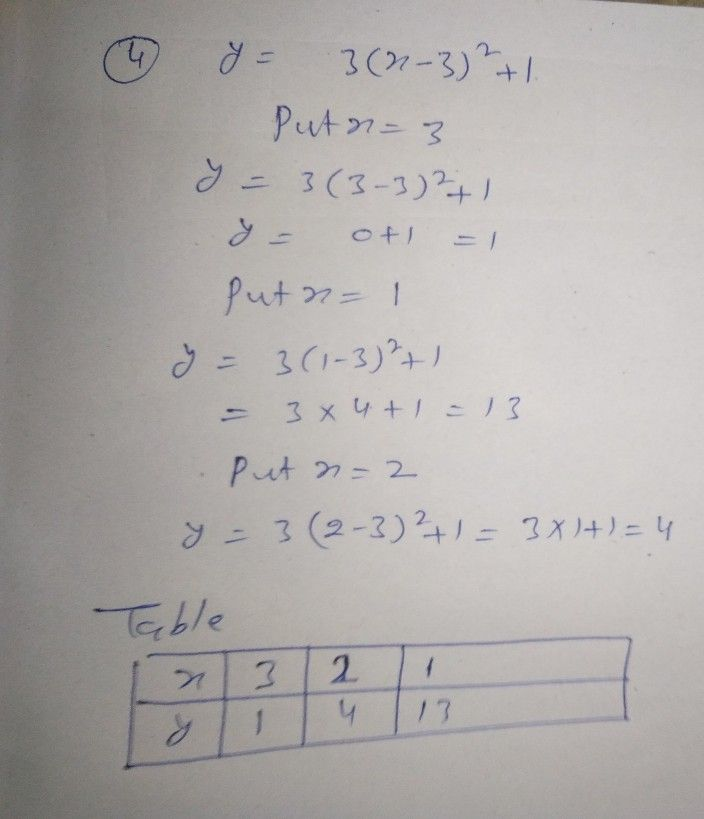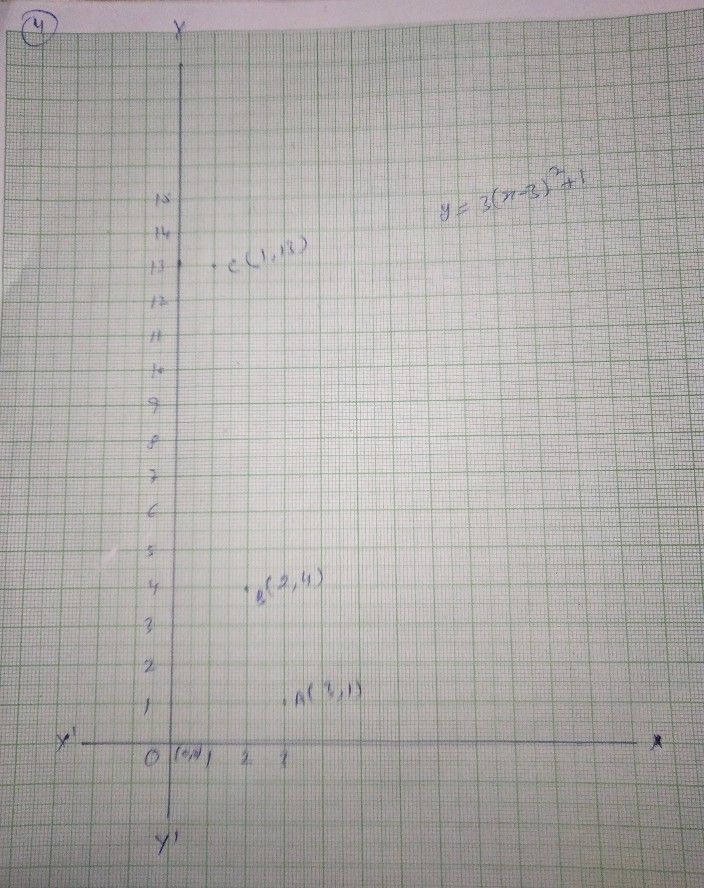Symbol
ProblemLearning Task $4$ Illustrate the graphs of the following quadratic functions then analyze the effects from each other. $1.y=3x2$ $2.y=3x2-3$ $3.y=3x2+3$ $4.y=3\left(x-3\right)^{2+1}$ $2$ $2$ $\bar{2}$ $\bar{1}$ $5.y=3\left(x+3\right)2-1$ $3$ $Explanation:$
7th-9th grade
Trigonometry
Search count: 106
SolutionQanda teacher - Lokesh